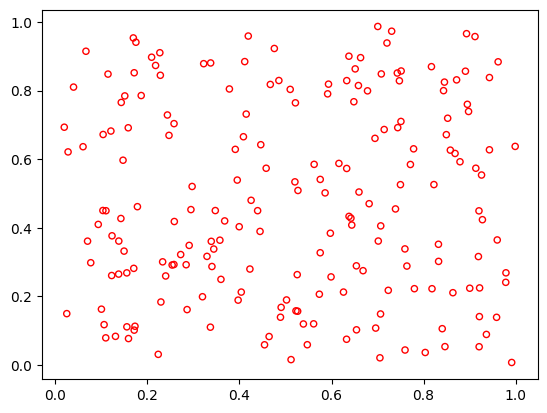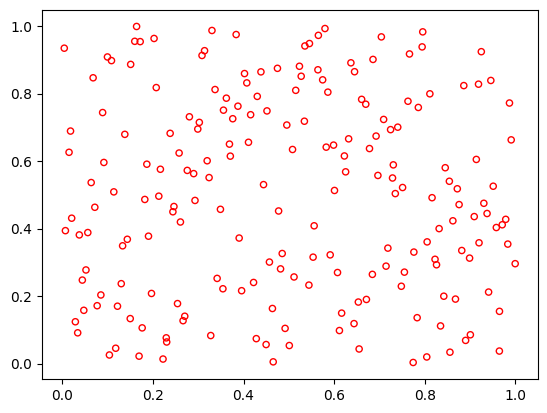Version: 0.5.0

# Sampling¶

In the beginning, initial points need to be sampled. pymoo offers different sampling methods depending on the variable type.

## Random Sampling (‘real_random’, ‘int_random’, ‘bin_random’)¶

:

from pymoo.factory import get_sampling
from pymoo.util import plotting
from pymoo.interface import sample

sampling = get_sampling('real_random')

X = sample(sampling, 100, 2)
plotting.plot(X, no_fill=True)## Latin Hypercube Sampling (‘real_lhs’)¶

:

from pymoo.factory import get_sampling
from pymoo.util import plotting
from pymoo.interface import sample

sampling = get_sampling('real_lhs')

X = sample(sampling, 100, 2)
plotting.plot(X, no_fill=True)## API¶

pymoo.factory.get_sampling(name, kwargs)

A convenience method to get a sampling object just by providing a string.

Parameters
name{ ‘real_random’, ‘real_lhs’, ‘bin_random’, ‘int_random’, ‘int_lhs’, ‘perm_random’ }

Name of the sampling.

kwargsdict

Dictionary that should be used to call the method mapped to the sampling factory function.

Returns
classSampling

An sampling object based on the string. None if the sampling was not found.

pymoo.core.sampling.Sampling()None